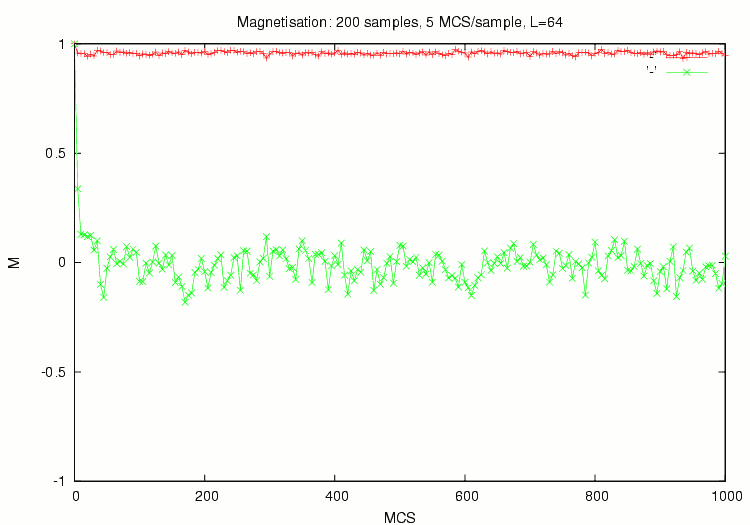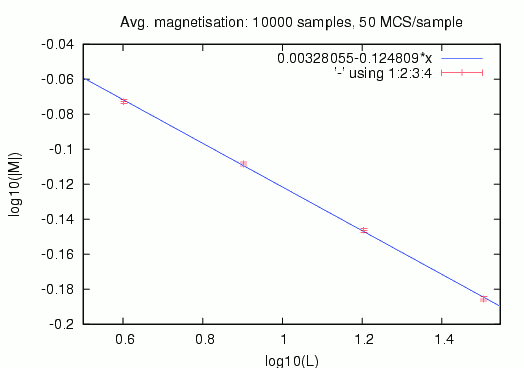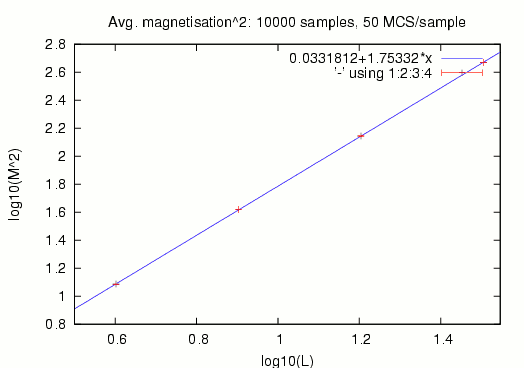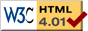Nav: [home] > [sim] > [isingmag]

## Simple Ising model magnetisation simulation

Simulation of Ising model in a quadratic 2d area of variable length with external magnetic field switched off (H=0). Nearest neighbour interaction is assumed (i.e. each spin has 4 neighbours); uses periodic boundary conditions.

### News

10/2005: Internal energy calculation implemented
Version 1.3 now also calculates the internal energy H (Hamiltonian) of the system; added graph showing the H versus temperature (v1.4) and finally also the heat capacity versus temperature can now be displayed (v1.5) showing the theoretically predicted divergence at TC.
Version 1.2 will now calculate the susceptibility versus temperature and show a steep divergence at the critical temperature.
08/2005: Initial Release
Initial release of the Ising model simulator including magnetisation graph.

### The Ising model

Imagine a quadratic 2d area with L2 spins on a grid. Each spin can either point up (+1) or down (-1). The average magnetisation of the area is the average spin value and hence between 1 (completely ordered state) and 0.

Neighbouring spins S and S' interact with an interaction energy of E=-JSS'. Since each spin has 4 nearest neighbours (periodic boundary conditions), the interaction energy per spin can be between -4J (all neighbours parallel to the center spin) and +4J (all 4 neighbours antiparallel to center spin) where J is the coupling strength. (There is no external magnetic field present.)

Generally, states with less energy are preferred, so the system stays in completely ordered state for zero temperature. However, as we incease temperature, each spin has a thermal energy of kBT (where kB is Boltzmann's constant and T is the absolute temperature). Due to this themal energy, the system does not stay in completely ordered state but spins start to flucutate ("flip") randomly.

There is a phase transition at the critical temperature of kBT/J=2.269185: Starting with a completely ordered state, the system stays mostly ordered below the critical temperature while it goes completely unordered above it. Hence, above the critical temperature, the average magnetisation is (about) zero while it is non-zero below it.

In the simulation, whenever flipping a spin lowers the interaction energy, the flip is done. If it increases the energy, the flip is only committed with a probability of exp(-ßE) where ß=1/kBT and E>0 is the energy difference between flipped and unflipped state (Metropolis algorithm). As one can see, the relevant temperature can be expressed in units of ßJ which is called the reduced temperature and is the "natural" temperature unit used throughout the implementation.

The simulation repeatedly computes so-called MCS (Monte-Carlo step), commonly also referred to as time, each of whom involves the potential flipping (as explained above) of all spins in the area. Usually, the average magnetisation is computed in regular intervals (e.g. every 20 MCS) and averaged over several samples to estimate the standard deviation of the magnetisation value.

### The simulation

For the actual simulation, I wrote a small program which does not require any additional libraries, just a C++ compiler and GNUPlot(1) for the graphics. It compiles natively under Linux but porting to other operating system should not be hard.

The full source code is provided here so that everybody can do his own experiments. For more information on the simulation and where to tune the parameters refer to the comments in the source code.

 Source: isingmag.tar.gz   [10kb gzipped source tarball] Version: 1.5a   (2005-10-05) Author: Wolfgang Wieser   (report bugs here) License: GNU GPL (Version 2) Requires: C++ (gcc recommended), gnuplot

The program does different things depending on which function is not commented out in the main() routine at the end of the isim.cc file. In order to run a specific sim, uncomment the corresponding function (you may add functions yourself!), tune the parameters in that function and then use make run to have the simulation started and get the resulting graph plotted using gnuplot.

Gnuplot will typically not display the graph on the screen but dump it into a postscript file which can be displayed e.g. using gv.

### Example: Relaxation from ordered state

Starting from a completely ordered state, this displays the average magnetisation over time (i.e. MCS) at a reduced temperature of 1.0/1.8 (red graph) and 1.0/2.8 (green graph). As one can see, the first case is below the critical temperature and the system stays mostly ordered while the second case is above the critical temperature and the system rapidly goes into completely unordered state with magnetisation fluctuating thermically around zero.This example was made with the above program using the function DisplayMagnetisationPerSpinOverTime():

```void DisplayMagnetisationPerSpinOverTime(
double betaJ_1,double betaJ_2)
{
int nsamples=200;        // <-- # of data points in diagram (time axis).
int mcs_per_sample=5;    // <-- Record a data point every this many MCS.
IsingSimulator sim(64);  // <-- Tune are size here.

const char *filename="magnetisation_per_spin_over_time.ps";

...snip...
}

int main()
{
// Choose what the program should simulate:
// Uncomment at least one line.
// Feel free to add further functions.

DisplayMagnetisationPerSpinOverTime(1.0/1.8, 1.0/2.8);
//DisplayMagnetisationTimeAverage();
//ComputeCriticalExponents();

return(0);
}
```

### Example: Magnetisation

The phase transition can most easily be seen when starting with a completely ordered state and computing the average magnetisation after lots of MCS for different temperatures. The below graph shows the absolute value of the average magnetisation versus reciprocal reduced temperature (kBT/J) from 1 to 4 for area sizes 32x32 (green) and 256x256 (violet).

As one can see, for zero temperature, the state stays completely ordered. As we increase temperature, the magnetisation starts to drop more and more rapidly until the phase transition. Above the critical temperature of about kBT/J=2.27, the absolute average magnetisation is nearly zero. The larger the area the more drastic the effect because for larger areas, thermal fluctuations cancel better when averaging.The above diagram was simulated with the function DisplayMagnetisationTimeAverage() included in the source code. Parameters:

```void DisplayMagnetisationTimeAverage()
{
// R_betaJ0 is 1.0/(beta * J).
double R_betaJ0=1.0;   // from here...
double R_betaJ1=4.0;   // ...to here in...

// Make the complete temperature sweep from R_betaJ0 to R_betaJ1 for
// each area size specified in this array. LAST ENTRY MUST BE -1.
int l_array[]={32,/*64,128,*/256,-1};
// Number of steps in the transition from R_betaJ0 to R_betaJ1:
// This has to be set for every area size.
int nsteps_array[]={21,/*21,61,*/61,-1};

// Never take more than this number of samples for the average:
int max_samples=1000;
// Do at least this many samples:
int min_samples=20;
// Stop taking samples if the estimate for the standard deviation
// is smaller than this:
double min_sigma=1e-3;
// A sample is taken after this many MCS:
int samp_mcs=20;
// Do this many MCS initially to equilibrate:
int neq_mcs=20;

const char *filename="magnetisation_vs_temperature.ps";

...snip...
}
```

### Example: Finite size scaling and critical exponents

By simulating the ising model at the critical temperature, for different area sizes, one can determine two ratios of critical exponents: beta/nu and gamma/nu. Basically, we sample the average magnetisation for many MCS to compute an average absolute magnetisation as well as an average squared magnetisation (both with standard deviation) for each area size. Then, these values get plotted in double-logarithmic diagrams (see below) and a linear fit is performed. Then, -beta/nu and gamma/nu are the slopes of the graphs, respectively.The linear fits are performed automatically and the program computes values with error: (There is a sanity check: 2*beta+gamma should be nu*d (with d=2 dimension of the area) which is computed in the "Test" line.)

```Linear fit: chi^2=2.45892; a=0.00328055±0.00188105, b=-0.124809±0.00171727
--> beta/nu=0.124809±0.00171727
Linear fit: chi^2=4.78236; a=0.0331812±0.0027958, b=1.75332±0.00257005
--> gamma/nu=1.75332±0.00257005
Test: 2.00294±0.00428967 (should be 2)
```

For the above results, the following function from the source code was used: (Don't forget to un-comment ComputeCriticalExponents(); in main().)

```void ComputeCriticalExponents()
{
// Number of magnetization samples to gather.
int nsamples=10000;
// Number of complete MCS to compute for each (of the nsamples) sample.
int mcs_per_sample=50;

// Use (finite) area sizes specified here in ascenting order.
// MUST BE TERMINATED BY -1.
int l_array[]={4,8,16,32,-1};

const char *filename="magnetisation_vs_area_size.ps";

...snip...
}
```

### Example: Susceptibility

The susceptibility (chi) is the derivative of the magnetisation (per spin) by the external magnetic field (dm/dH). According to the fluctuation dissipation theorem (FDT), this is the variance (squared standard deviation) of the average spin value and hence expresses the spin fluctuation around the mean value. According to the theory, this value should diverge at the phase transition for infinitely sized areas.
This simulation shows the steep increase of the susceptibility near the critical temperature (which is at 2.269).The above diagram was computed using these settings for DisplaySusceptibilitySweep(). However, note that the complete calculation time was nearly 4 hours on a P4.

```void DisplaySusceptibilitySweep()
{
double R_betaJ0=1;   // from here...  2.1
double R_betaJ1=4;   // ...to here in...  2.7
int l_array[]={/*32,64,128,*/256,-1};
int nsteps_array[]={/*21,21,61,*/201,-1};

int max_samples=300000;
int min_samples=20;
double min_sigma=10;
int samp_mcs=20;
int neq_mcs=20;

const char *filename="chi_vs_temperature.ps";
const char *datafile="suscept.dat";

...snip...
}
```

### Example: Internal energy

The internal energy of the system is its Hamiltonian H. For better comparability of different system sizes, the internal energy per spin H/N is better suited. In natural units, the completely ordered phase has H/N=-2 since each pair of equally oriented spins contributes a value of -1 to the energy sum. Below is a sweep of the internal energy per spin over temperature for three different area sizes.This diagram was computed using the following parameters for DisplayInternalEnergySweep(). The calculation time is about a minute on an average AthlonXP.

```void DisplayInternalEnergySweep()
{
double R_betaJ0=1;   // from here...
double R_betaJ1=4;   // ...to here in...
int l_array[]={8,32,128,-1};
int nsteps_array[]={61,61,61,-1};

int max_samples=10000;
int min_samples=20;
double min_sigma=5e-3;
int samp_mcs=20;
int neq_mcs=20;

const char *filename="h_vs_temperature.ps";
const char *datafile="hamilton.dat";

...snip...
}
```

### Example: Heat capacity

According to the fluctuation dissipation theorem, the heat capacity (CV) is basically the variation of the energy (i.e. the Hamiltonian) of the system divided by the square of the temperature (CV/kB2Var(H)=Var(ßH)). For improved comparability among different system sizes, it is better to compute the heat capacity per spin (CV/NkB) which is displayed in the following graph.

The heat capacity also diverges at the phase transition not as drastically as the susceptibility (see above). According to theoretical derivations, the heat capacity should behave like log(|T-TC|) near the critical temperature; see the (manually fitted) cyan-colored curve. (Note that due to finite size effects, the peak is flattened and moved to the right. The only free parameter in the fit was the Y scale; when additionally fitting offset and critical temperature, a better overlap could be achieved.)The above diagram is comopsed from two calls to DisplayHeatCapacitySweep(); note that it took me over an hour simulation time on an average AthlonXP.

```void DisplayHeatCapacitySweep()
{
double R_betaJ0=1;   // from here...
double R_betaJ1=4;   // ...to here in...
int l_array[]={16,64/*,128,256*/,-1};
int nsteps_array[]={61,61/*,21,41,61*/,-1};

int max_samples=10000;
int samp_mcs=20;
int neq_mcs=20;

const char *filename="cv_vs_temperature.ps";
const char *datafile="heatcap.dat";

// Second call for more details in the critical region:
double R_betaJ0=2.2;
double R_betaJ1=2.35;
int l_array[]={/*16,*/64/*,128,256*/,-1};
int nsteps_array[]={/*61,*/10/*,21,41,61*/,-1};
}
```